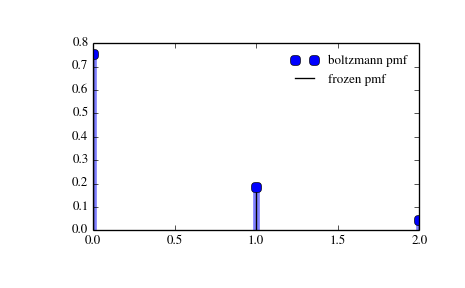# scipy.stats.boltzmann¶

scipy.stats.boltzmann = <scipy.stats._discrete_distns.boltzmann_gen object at 0x2b45d304a710>[source]

A Boltzmann (Truncated Discrete Exponential) random variable.

Discrete random variables are defined from a standard form and may require some shape parameters to complete its specification. Any optional keyword parameters can be passed to the methods of the RV object as given below:

Parameters: x : array_like quantiles q : array_like lower or upper tail probability lambda_, N : array_like shape parameters loc : array_like, optional location parameter (default=0) size : int or tuple of ints, optional shape of random variates (default computed from input arguments ) moments : str, optional composed of letters [‘mvsk’] specifying which moments to compute where ‘m’ = mean, ‘v’ = variance, ‘s’ = (Fisher’s) skew and ‘k’ = (Fisher’s) kurtosis. (default=’mv’) Alternatively, the object may be called (as a function) to fix the shape and location parameters returning a “frozen” discrete RV object: rv = boltzmann(lambda_, N, loc=0) Frozen RV object with the same methods but holding the given shape and location fixed.

Notes

The probability mass function for boltzmann is:

```boltzmann.pmf(k) = (1-exp(-lambda_)*exp(-lambda_*k)/(1-exp(-lambda_*N))
```

for k = 0,..., N-1.

boltzmann takes lambda_ and N as shape parameters.

Examples

```>>> from scipy.stats import boltzmann
>>> import matplotlib.pyplot as plt
>>> fig, ax = plt.subplots(1, 1)
```

Calculate a few first moments:

```>>> lambda_, N = 1.4, 19
>>> mean, var, skew, kurt = boltzmann.stats(lambda_, N, moments='mvsk')
```

Display the probability mass function (pmf):

```>>> x = np.arange(boltzmann.ppf(0.01, lambda_, N),
...               boltzmann.ppf(0.99, lambda_, N))
>>> ax.plot(x, boltzmann.pmf(x, lambda_, N), 'bo', ms=8, label='boltzmann pmf')
>>> ax.vlines(x, 0, boltzmann.pmf(x, lambda_, N), colors='b', lw=5, alpha=0.5)
```

Alternatively, freeze the distribution and display the frozen pmf:

```>>> rv = boltzmann(lambda_, N)
>>> ax.vlines(x, 0, rv.pmf(x), colors='k', linestyles='-', lw=1,
...         label='frozen pmf')
>>> ax.legend(loc='best', frameon=False)
>>> plt.show()
```Check accuracy of cdf and ppf:

```>>> prob = boltzmann.cdf(x, lambda_, N)
>>> np.allclose(x, boltzmann.ppf(prob, lambda_, N))
True
```

Generate random numbers:

```>>> r = boltzmann.rvs(lambda_, N, size=1000)
```

Methods

 rvs(lambda_, N, loc=0, size=1) Random variates. pmf(x, lambda_, N, loc=0) Probability mass function. logpmf(x, lambda_, N, loc=0) Log of the probability mass function. cdf(x, lambda_, N, loc=0) Cumulative density function. logcdf(x, lambda_, N, loc=0) Log of the cumulative density function. sf(x, lambda_, N, loc=0) Survival function (1-cdf — sometimes more accurate). logsf(x, lambda_, N, loc=0) Log of the survival function. ppf(q, lambda_, N, loc=0) Percent point function (inverse of cdf — percentiles). isf(q, lambda_, N, loc=0) Inverse survival function (inverse of sf). stats(lambda_, N, loc=0, moments=’mv’) Mean(‘m’), variance(‘v’), skew(‘s’), and/or kurtosis(‘k’). entropy(lambda_, N, loc=0) (Differential) entropy of the RV. expect(func, lambda_, N, loc=0, lb=None, ub=None, conditional=False) Expected value of a function (of one argument) with respect to the distribution. median(lambda_, N, loc=0) Median of the distribution. mean(lambda_, N, loc=0) Mean of the distribution. var(lambda_, N, loc=0) Variance of the distribution. std(lambda_, N, loc=0) Standard deviation of the distribution. interval(alpha, lambda_, N, loc=0) Endpoints of the range that contains alpha percent of the distribution

#### Previous topic

scipy.stats.binom

#### Next topic

scipy.stats.dlaplace